# Linear Impulse Momentum Relations (Part - 3) Civil Engineering (CE) Notes | EduRev

## Civil Engineering (CE) : Linear Impulse Momentum Relations (Part - 3) Civil Engineering (CE) Notes | EduRev

The document Linear Impulse Momentum Relations (Part - 3) Civil Engineering (CE) Notes | EduRev is a part of the Civil Engineering (CE) Course Introduction to Dynamics and Vibrations- Notes, Videos, MCQs.
All you need of Civil Engineering (CE) at this link: Civil Engineering (CE)

Example 2: Two frictionless spheres with radius R have initial velocity vA0 vB0 . At some instant of time, the two particles collide. At the point of collision, the centers of the spheres have position vectors yA yB . The restitution coefficient for the contact is denoted by e. Find a formula for the velocities of the spheres after impact. Hence, deduce an expression for the change in kinetic energy during the impact.

This is a straightforward vector algebra exercise. We have two unknown velocity vectors: vA1 vB1 , and two vector equations – momentum conservation, and the restitution coefficient formula.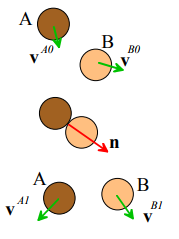Calculation

1. Note that a unit vector normal to the tangent plane can be calculated from the position vectors of the centers at the impact as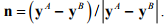It doesn’t matter whether you choose to take n to point from A to B or the other way around – the formula will work either way.

2. Momentum conservation requires that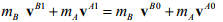3. The restitution coefficient formula gives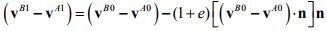4. We can solve (2) and (3) for vB1 by multiplying (3) by mA and adding the equations, which gives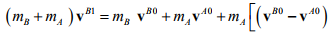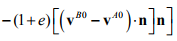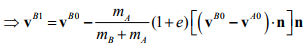5. Similarly, we can solve for vA1 by multiplying (3) by mB and subtracting (3) from (2), with the result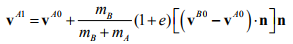6. The change in kinetic energy during the collision can be calculated as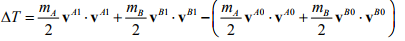7. Substituting the results of (4) and (5) for vB1 and vA1 and simplifying the result gives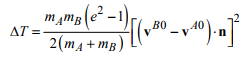Note that the energy change is zero if e=1 (perfectly elastic collisions) and is always negative for e<1 (i.e. the kinetic energy after collision is less than that before collision).

Example 3: This is just a boring example to help illustrate the practical application of the vector formulas in the preceding example. In the figure shown, disk A has a vertical velocity V at time t=0, while disk B is stationary. The two disks both have radius R, have the same mass, and the restitution coefficient between them is e. Gravity can be neglected. Calculate the velocity vector of each disk after collision.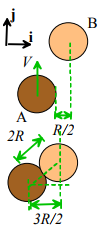Calculation

1. Before impact, the velocity vectors are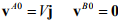2. A unit vector parallel to the line joining the two centers is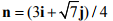(to see this, apply Pythagoras theorem to the triangle shown in the figure).

3. The velocities after impact are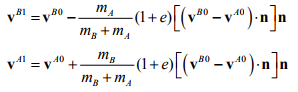Substituting the vectors gives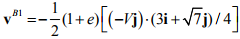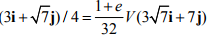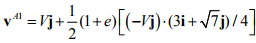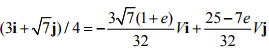Example 4: How to play pool (or snooker, billiards, or your own favorite bar game involving balls, a stick, and a table…). The figure shows a typical problem faced by a pool player – where should the queue ball hit the eight ball to send it into the pocket?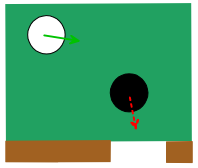This is easily solved – the eight ball is stationary before impact, and after impact has a velocity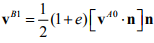Notice that the velocity is parallel to the unit vector n. This vector is parallel to a line connecting the centers of the two balls at the instant of impact. So the correct thing to do is to visualize an imaginary ball just touching the eight ball, in line with the pocket, and aim the queue ball at the imaginary ball. Easy!

The real secret to being a successful pool player is not potting the balls – that part is easy. It is controlling where the queue ball goes after impact. You may have seen experts make a queue ball reverse its direction after an impact (appearing to bounce off the stationary ball); or make the queue ball follow the struck ball after the impact. According to the simple equations developed here, this is impossible – but a pool table is more complicated, because the balls rotate, and are in contact with a table. By giving the queue ball spin, an expert player can move the queue ball around at will. To make the ball rebound, it must be struck low down (below the ‘center of percussion’) to give it a reverse spin; to make it follow the struck ball, it should be struck high up, to make it roll towards the ball to be potted. Giving the ball a sideways spin can make it rebound in a controllable direction laterally as well. And it is even possible to make a queue ball travel in a curved path with the right spin.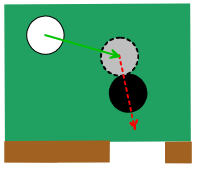Never let it be said that you don’t learn useful skills in engineering classes!

Example 5: In this problem we set up a simple MATLAB program that analyzes motion with impacts. The problem to be solved in illustrated in the figure. A square box contains a number of rigid, frictionless circular disks. The disks all have the same mass. At time t=0 the disks are given some random distribution of velocities. The goal of the program is to compute and animate the subsequent motion of the disks, accounting for all collisions.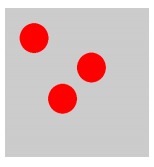To do this, we need to:

1. Derive the equations of motion for each disk and integrate them;
2. Detect collisions between particles and the wall, and change the particle velocity to account for the collision;
3. Detect collisions between two particles, and change the velocities of both colliding particles to account for the collision.

We will model the system by calculating the (x,y) coordinates of each disk. In the following, we will use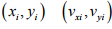to denote the position and velocity of the ith disk.

Equations of motion : No forces act on the disks except during the collisions. Therefore, between collisions, the equations of motion for the ith disk is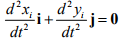As always, we turn this into MATLAB form by writing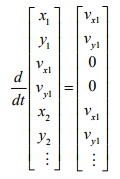Collisions with the walls: The collisions occur at the instants when the distance of a disk to the wall is equal to the radius of the disk. Therefore

1. Collision with the wall at x=L occurs when L - xi - R = 0
2. Collision with the wall at x=-L xi - R + L = 0
3. Collision with the roof at y=L occurs when L - yi - R = 0
4. Collision with the floor at y=-L occurs when yi - R + L = 0

A collision can be detected by detecting the point when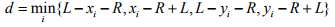crosses zero from above.

When a collision occurs, the velocities must be corrected as follows to account for the collision:

1. Collision with the walls at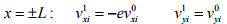2. Collision with the walls at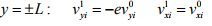Collisions between particles The collisions occur at the instants when the distance between the centers of any two disks is equal to the diameter of the disk. When a collision occurs between the ith and jth particles, their velocities must be corrected as follows to account for the collision: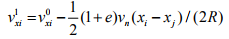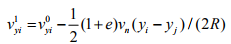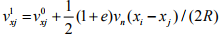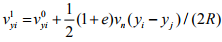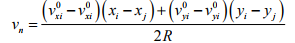To see this, note that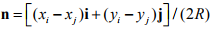is a unit vector joining the two centers; and  let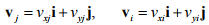denote the velocities of the two particles, and substitute into the general equations in Example 4.

Here is a MATLAB script that implements these calculations. It will produce the animation shown in the picture. You can make various modifications to solve similar problems. The only new feature of this code that has not been discussed before is the ODE solver – it turns out that the standard solver ode45 is not accurate enough for this problem and misses some collisions. The more accurate solver ode113 is used instead.

function disk_collisions
% Function to compute and animate motion of colliding disks
% in a box
n_disks = 3;
box_size = 10.0;
restitution_wall = 1;
restitution_disk = 1;
% These variables are defined in the outer function so they are available
% in all functions
disk_wall_index = 1; %specifies the disk that is closest to a wall
colliding_pair(1) = 1; %specifies first disk in pair of closest disks
colliding_pair(2) = 1; %specifies second disk in pair of closest disks
tstart = 0;
tstop = 60;
count = 0;
y0 = [1.,1.,0.,0.,-1.,-1.1,1.,1.,-3.,3.,-0.25,0.25];

while(tstart<stop)
Abstol = 10^(-05)*ones(4*n_disks,1);
options =
odeset('RelTol',0.0000001,'AbsTol',Abstol,'Events',@detect_collision);
[t,y,tevent,yevent,ievent] = ode113(@eom,[tstart,tstop],y0,options);

if (~isempty(tevent))
y0 = collide(tevent,yevent,ievent);
end

tstart = t(length(t));
for i=1:length(t)
% The disks are a single point on an x-y plot
hold off
plot(y(i,1),y(i,2),'ro','MarkerSize',52,'MarkerFaceColor','r');
hold on
for n = 2:n_disks
plot(y(i,4*(n-1)+1),y(i,
4*(n-1)+2),'ro','MarkerSize',52,'MarkerFaceColor','r');
end
axis([-5,5,-5,5]);
axis square
pause(0.025) % This just slows down the animation a bit
end

end

function dydt = eom(t,y)
% Equations of motion for the disks
% y contains [x,y,vx,vy,…] for each disk
for n=1:n_disks
i = 4*(n-1)+1;
dydt(i) = y(i+2);
dydt(i+1) = y(i+3);
dydt(i+2) = 0;
dydt(i+3) = 0.;

end
dydt = transpose(dydt);

end

function [eventvalue,stopthecalc,eventdirection] = detect_collision(t,y)
% Function to detect a collision of a disk with a wall or another disk
%
% Find the shortest distance between a disk and a wall
dmin = box_size;
for n=1:n_disks
i = 4*(n-1)+1;
[d,min_wall] = min([d1,d2,d3,d4]);
if (d<dmin)
dmin = d;
disk_wall_index = n;
end
end
% First event is collision of disk with a wall
eventvalue(1) = dmin;
stopthecalc(1) = 1;
eventdirection(1)=-1;
dmin = 10*box_size;
% Find the min dist between any pair of disks
if (n_disks>1)
for n=1:n_disks-1
i = 4*(n-1)+1;
for m = n+1:n_disks
j = 4*(m-1)+1;
d = sqrt( (y(j)-y(i))^2 + (y(j+1)-y(i+1))^2)-2*disk_radius;
if (d<dmin)
dmin = d;
colliding_pair(1)=n;
colliding_pair(2)=m;
end
end
end
% Second event is collision of two disks
eventvalue(2) = dmin;
stopthecalc(2) = 1;
eventdirection(2)=-1;
end
end
function y0 = collide(t,y,ievent)
% Function to change velocity of disks when a collision occurs

y0 = y;

if (ievent==1) %collision with a wall
i = 4*(disk_wall_index-1)+1;
if (d1<0.0001 || d2<0.0001) %collision with left or right wall
y0(i+2) = -restitution_wall*y0(i+2);
end
if (d3<0.0001 || d4<0.0001) % collision with top or bottom wall
y0(i+3) = -restitution_wall*y0(i+3);
end
else
i = 4*(colliding_pair(1)-1)+1;
j = 4*(colliding_pair(2)-1)+1;
vn = (y(j+2)-y(i+2))*normal(1) + (y(j+3)-y(i+3))*normal(2);
y0(i+2) = y0(i+2) + (1+restitution_disk)*vn*normal(1)/2;
y0(i+3) = y0(i+3) + (1+restitution_disk)*vn*normal(2)/2;
y0(j+2) = y0(j+2) - (1+restitution_disk)*vn*normal(1)/2;
y0(j+3) = y0(j+3) - (1+restitution_disk)*vn*normal(2)/2;
end
end
end

Offer running on EduRev: Apply code STAYHOME200 to get INR 200 off on our premium plan EduRev Infinity!

## Introduction to Dynamics and Vibrations- Notes, Videos, MCQs

20 videos|53 docs

,

,

,

,

,

,

,

,

,

,

,

,

,

,

,

,

,

,

,

,

,

;